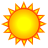# Convert DEM pixels to points

4586
5
06-04-2013 07:01 AMNew Contributor
Hi all,

I'm following a tutorial to calculate spatially explicit hypsometric integral values. The first step is described as follows:

"1.) Zpixel = Elevation of a pixel
Convert DEM pixels to points. VALUE field in attribute table contains elevations"

I'm not sure which tool to use to achieve this conversion. The DEM of my watershed is a 32 bit floating point GRID raster. I tried the Spatial Analyst Tool, Extract Values to Points, using two blank point shapefiles, but the output point shapefile remained blank. I don't understand what information is needed for the "Input point shapefile" field; again, I tried a blank point shapefile in that field to no avail. Any ideas?
Tags (3)
1 Solution

Accepted SolutionsRegular Contributor III
but you will find that it doesn't allow floating point input

Eric - Using v10.1 SP1 with a 32-bit float (grid format) as input. Points correctly get a GRID_CODE field of type Float containing floating point values (example: -1.0675).
5 RepliesRegular Contributor IIIbyEsri Regular Contributor
Raster to Point is the tool to use, but you will find that it doesn't allow floating point input.  It is required that an integer raster be input.  You can run the Int tool to truncate the decimals, or if you need to retain the decimals then multiply the raster (Times tool) by 10, 100, 1000 etc... depending on how many digits to the right of the decimal you wish to maintain.  After multiplying, run Int, then convert to points.  In the point attribute table you should see the Value field (sometimes called GRID_CODE) that stores the raster value.  You can use the field calculator to divide by 10, 100, 1000 to get your decimals back.

The other tools you mention require you have existing points and you sample the DEM using those points.  So if you don't want to fuss with multiplying and field calculations, you can run Int tool, then convert to points, then use those points to sample the original 32 bit floating point DEM.  i.e. Extact Values to Points, Sample, etc..

Best,
EricRegular Contributor III
but you will find that it doesn't allow floating point input

Eric - Using v10.1 SP1 with a 32-bit float (grid format) as input. Points correctly get a GRID_CODE field of type Float containing floating point values (example: -1.0675).New Contributor
Eric and Chris:

Thanks for your help. I was able to successfully convert the 32-bit floating point raster to a point shapefile, using the Raster to Point tool in ArcGIS 10.0. The elevation values for each pixel have been assigned to the GRID_CODE attribute in the point shapefile. I should've known to look under Conversion Tools, but my GIS is a bit rusty.byEsri Regular Contributor
You sir are correct.  It's the Raster to Polygon and Raster to Polyline that are strictly integer/string input.  Thanks for catching my mistake!

So, just run Raster to Point...

Best,
Eric